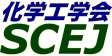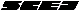## \$B9V1i?=\$79~\$_0lMw!J%-!<%o!<%IJL!K(B

\$B:G=*99?7F|;~!'(B2012-09-29 21:47:01

\$B:G=i\$NJ8;z(B\$B%-!<%o!<%INc(B\$B1d\$Y7o?t(B
0-913C-Metabolic flux analysis (1 \$B7o(B), 1-Dodecanol (1 \$B7o(B), 2-Methacryloyloxyethyl phosphorylcholine polymer (1 \$B7o(B), 2-methoxyethylacrylate (1 \$B7o(B), 2-fluid model equation (1 \$B7o(B), 2-propanol dehydration (1 \$B7o(B) \$BEy(B7 \$B7o(BAadsorption (19 \$B7o(B), ammonia (9 \$B7o(B), amine (5 \$B7o(B), Atmospheric plasma (4 \$B7o(B), activated carbon (4 \$B7o(B), anode (3 \$B7o(B) \$BEy(B174 \$B7o(BBBiomass (19 \$B7o(B), bioethanol (5 \$B7o(B), biorefinery (4 \$B7o(B), Biosensor (3 \$B7o(B), Biomass Tar (2 \$B7o(B), bioinformatics (2 \$B7o(B) \$BEy(B105 \$B7o(BC-CHCarbon Dioxide (9 \$B7o(B), chemical vapor deposition (8 \$B7o(B), CFD (6 \$B7o(B), cake characteristics (5 \$B7o(B), CCS (5 \$B7o(B), cerium oxide (4 \$B7o(B) \$BEy(B165 \$B7o(BCI-CZcrystallization (17 \$B7o(B), CVD (10 \$B7o(B), Crystal structure (9 \$B7o(B), coating (5 \$B7o(B), classification (5 \$B7o(B), cooling crystallization (4 \$B7o(B) \$BEy(B208 \$B7o(BDDEM (5 \$B7o(B), dielectrophoresis (4 \$B7o(B), Draft tube (4 \$B7o(B), density (4 \$B7o(B), droplet (3 \$B7o(B), Drug Delivery System (3 \$B7o(B) \$BEy(B142 \$B7o(BEextraction (10 \$B7o(B), electrodeposition (5 \$B7o(B), Electrolyte (5 \$B7o(B), energy saving (4 \$B7o(B), Escherichia coli (3 \$B7o(B), enzyme (3 \$B7o(B) \$BEy(B120 \$B7o(BFFuel cell (8 \$B7o(B), fluidized bed (6 \$B7o(B), fouling (5 \$B7o(B), filtration (3 \$B7o(B), FCC (2 \$B7o(B), filtration pressure (2 \$B7o(B) \$BEy(B91 \$B7o(BGGasification (6 \$B7o(B), Graphene (5 \$B7o(B), Grinding (3 \$B7o(B), growth rate (2 \$B7o(B), glucose (2 \$B7o(B), gene delivery (2 \$B7o(B) \$BEy(B82 \$B7o(BHhydrogel (6 \$B7o(B), hydroxyapatite (6 \$B7o(B), heat pump (5 \$B7o(B), hydrolysis (5 \$B7o(B), high pressure (4 \$B7o(B), hydrogen (4 \$B7o(B) \$BEy(B153 \$B7o(BIionic liquid (15 \$B7o(B), ice templating method (4 \$B7o(B), Information technology (2 \$B7o(B), ion exchange resin (2 \$B7o(B), Indium (2 \$B7o(B), Incineration ash (2 \$B7o(B) \$BEy(B90 \$B7o(BKKinetics (2 \$B7o(B), kidney (1 \$B7o(B), Kyodai nitration (1 \$B7o(B), kinematics of fluidized objects (1 \$B7o(B), Kenics Static Mixer (1 \$B7o(B), K-loaded char (1 \$B7o(B) \$BEy(B14 \$B7o(BLLiposome (8 \$B7o(B), lactic acid (5 \$B7o(B), Lorentz force (3 \$B7o(B), Luminescence (3 \$B7o(B), Liquid-liquid extraction (2 \$B7o(B), Layered double hydroxide (2 \$B7o(B) \$BEy(B102 \$B7o(BM-MHMembrane (12 \$B7o(B), Mesoporous silica (4 \$B7o(B), metallic bonding (2 \$B7o(B), Methane fermentation (2 \$B7o(B), Maintenance (2 \$B7o(B), Methane decomposition (2 \$B7o(B) \$BEy(B110 \$B7o(BMI-MZmicroreactor (12 \$B7o(B), MOC (9 \$B7o(B), mixing (7 \$B7o(B), microbubble (6 \$B7o(B), microfiltration (6 \$B7o(B), Microcapsule (6 \$B7o(B) \$BEy(B150 \$B7o(BNNanoparticle (16 \$B7o(B), Numerical simulation (9 \$B7o(B), nanoparticles (8 \$B7o(B), non solvent reaction (2 \$B7o(B), NOx (2 \$B7o(B), nanostructure (2 \$B7o(B) \$BEy(B96 \$B7o(BOoptimization (3 \$B7o(B), Ozonation (2 \$B7o(B), ontology (2 \$B7o(B), oxidation (1 \$B7o(B), Observation noise (1 \$B7o(B), Oxygen Mass transfer (1 \$B7o(B) \$BEy(B44 \$B7o(BP-PHphotocatalyst (8 \$B7o(B), PEFC (5 \$B7o(B), pervaporation (3 \$B7o(B), photovoltaic power generation (3 \$B7o(B), peptide (3 \$B7o(B), phase separation (3 \$B7o(B) \$BEy(B88 \$B7o(BPI-PZprocess intensification (5 \$B7o(B), plasma (3 \$B7o(B), protein (3 \$B7o(B), pressure drop (2 \$B7o(B), polymerization (2 \$B7o(B), protein purification (2 \$B7o(B) \$BEy(B146 \$B7o(BQQSPR (2 \$B7o(B), quality (2 \$B7o(B), QMS (2 \$B7o(B), QCM (2 \$B7o(B), quinoline (1 \$B7o(B), quick-boiling (1 \$B7o(B) \$BEy(B14 \$B7o(BRRecycling (4 \$B7o(B), rare earth (3 \$B7o(B), RESS method (3 \$B7o(B), reactor (3 \$B7o(B), recovery (2 \$B7o(B), Recycle (2 \$B7o(B) \$BEy(B98 \$B7o(BS-SOSimulation (11 \$B7o(B), solubility (8 \$B7o(B), SOFC (6 \$B7o(B), solid oxide fuel cell (5 \$B7o(B), silica (5 \$B7o(B), separation (5 \$B7o(B) \$BEy(B177 \$B7o(BSP-SZsupercritical carbon dioxide (10 \$B7o(B), supercritical water (10 \$B7o(B), surface modification (6 \$B7o(B), subcritical water (6 \$B7o(B), suspension (5 \$B7o(B), steam reforming (5 \$B7o(B) \$BEy(B159 \$B7o(BTthin film (4 \$B7o(B), Thermal energy storage (3 \$B7o(B), titanium dioxide (3 \$B7o(B), thermal plasma (3 \$B7o(B), Thermodynamic consistency line (3 \$B7o(B), TiO2 (2 \$B7o(B) \$BEy(B98 \$B7o(BUultrasonic (2 \$B7o(B), Ultrasound (2 \$B7o(B), Unidirectional freezing (1 \$B7o(B), ultrasonic hone (1 \$B7o(B), UNIQUAC equation (1 \$B7o(B), urease (1 \$B7o(B) \$BEy(B27 \$B7o(BVviscosity (7 \$B7o(B), vapor-liquid equilibria (4 \$B7o(B), VOC (3 \$B7o(B), visualization (3 \$B7o(B), Vesicle (3 \$B7o(B), vapor liquid equilibrium (2 \$B7o(B) \$BEy(B48 \$B7o(BWwaste concrete (3 \$B7o(B), whey (2 \$B7o(B), Woody Biomass (2 \$B7o(B), Water treatment (2 \$B7o(B), water purification (2 \$B7o(B), waste water treatment (2 \$B7o(B) \$BEy(B45 \$B7o(BXXylitol (1 \$B7o(B)1 \$B7o(BYyeast (2 \$B7o(B), Y type zeolite (1 \$B7o(B), Y-type zeolite (1 \$B7o(B)4 \$B7o(BZzeolite membrane (4 \$B7o(B), Zeolite (4 \$B7o(B), zeolite rho (2 \$B7o(B), zeta potential (2 \$B7o(B), Zinc oxide (2 \$B7o(B), zincoaluminosilicate (1 \$B7o(B) \$BEy(B27 \$B7o(B\$BHs1Q?t;z(B- (5 \$B7o(B), \$B@PC:(B (2 \$B7o(B), \$BG.J,2r(B (2 \$B7o(B), \$B%,%92=(B (2 \$B7o(B), \$B%P%\$%*%^%9(B (2 \$B7o(B), \$BH?1~7PO)(B (1 \$B7o(B) \$BEy(B56 \$B7o(B\$B
\$B2=3X9)3X2q(B \$BBh(B44\$B2s=)5(Bg2q(B(C) 2012 \$B8x1W
Most recent update: 2012-09-29 21:47:01
For more information contact \$B2=3X9)3X2qElKL;YIt(B \$BBh(B44\$B2s=)5(Bg2q(B \$BLd\$\$9g\$;78(B
E-mail: inquiry-44fwww3.scej.org
This page was generated byeasp 2.28; update.pl 2.28a (C)1999-2011 kawase